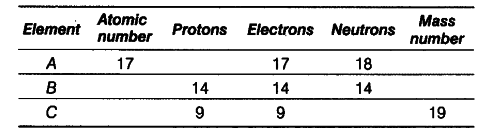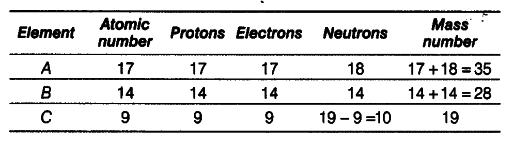# Complete following table:

Complete the following table.A: Atomic number = Number of protons =17;
Mass number = Number of protons + number of neutrons
= 17 + 18 = 35
B: Atomic number = Number of protons = 14
Mass number = Number of protons + number of neutrons = 14 + 14 = 28
C: Atomic number = number of protons = 9
Number of neutrons = Mass number - number of protons = 19-9 = 10